# How to Fill a Sequence Number and Repeat Them in Excel

In our work we often enter a sequence of numbers as ID for statistic. So we need to require a convenient way to enter the numbers by sequence. This article will introduce you a convenient way to fill numbers by custom, and repeat the sequence number by two ways.

## Fill a Sequence of Numbers

It is very easy if we want to enter a sequence of numbers like 1,2,3,4, we can directly enter 1 and 2 into first two columns or rows, then just need to drag them to fill the following cells, excel will auto fill the cells with the increase of value 1. If we want to enter the first number which is not started with 1, and the step value is not 1 nether, how can we create the numbers? You can learn the way by following steps.

Step 1: If we want to enter a sequence of numbers like 5,10,15,20, 25… till 100. First enter the started number 5 into A1 cell. Then click on Home tab.Step 2: Click on Fill button which is displayed in the right.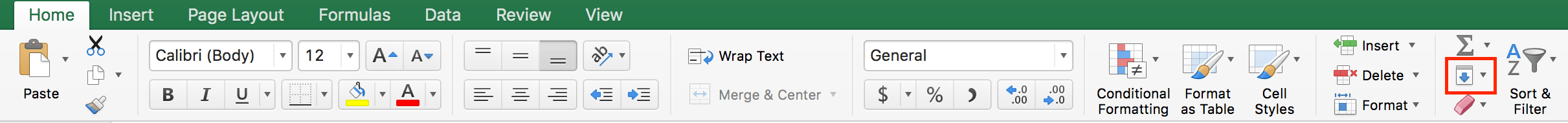Step 3: Click on the arrow on the button. Then select Series.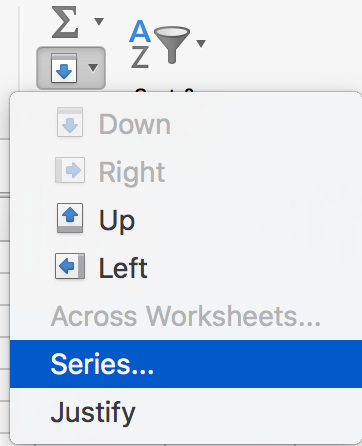Step 4: In Series, select Series in as Column, Type as Linear, Step value as 5, Stop value as 100. Then click OK.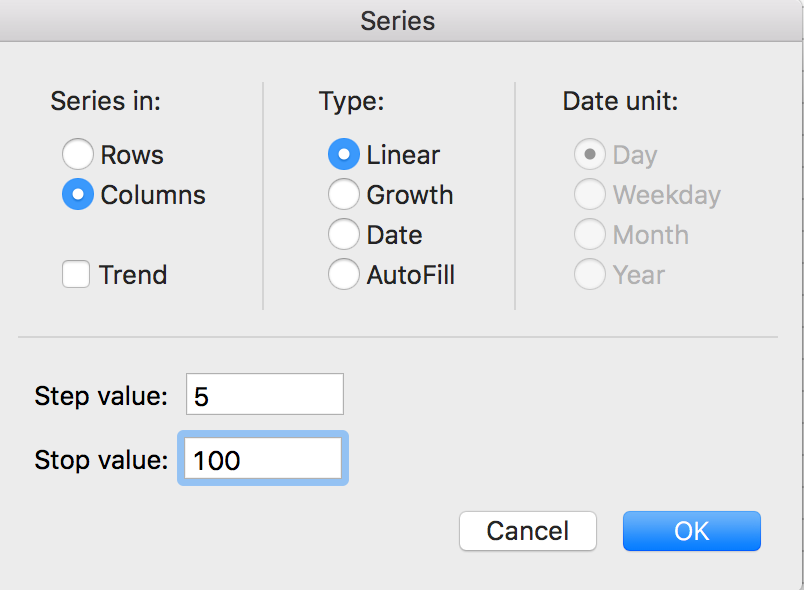Step 5: After above operation, we can find that A2 to A20 are filled with numbers 10, 15, 20…till 100. In A1 the start number is 5, in A20 the end number is 100. Between 5-100, number is increased with 5.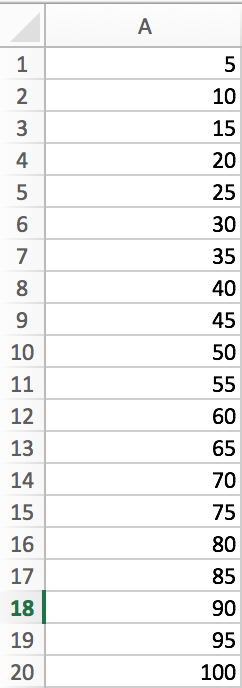## Repeat a Sequence of Numbers by Copy Cell

Step 1: Enter a sequence number for example 1,2,3,4 in excel A Column: A1-A4.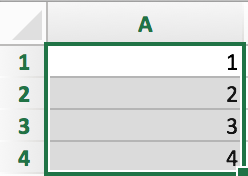Step 2: Drag it down and fill the cells in A column, then release the mouse. For example, we drag it to A12 in this case. Verify that after releasing the mouse, Auto Fill Options icon displays at the lower right corner of A12. And A5-A12 are filled with numbers 5-12 which are not what we expect.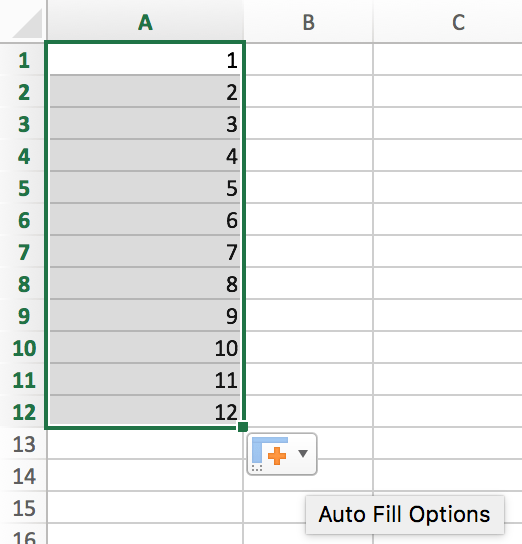Step 3: Click on Auto Fill Options. Check on Copy Cells option.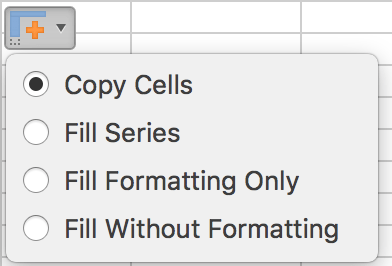Step 4: Now A5-A12 cells are filled with 1,2,3,4 repeatedly.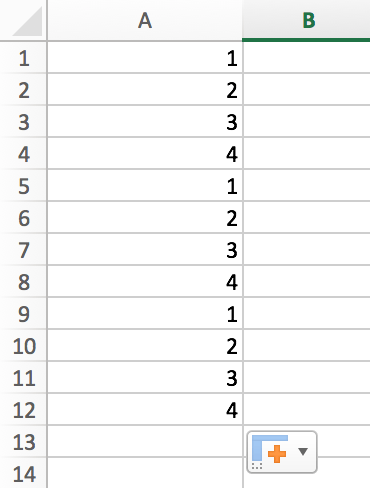## Repeat a Sequence of Numbers by Formula

We want get a sequence number like 1,2,3,4,1,2,3,4 etc. Here we can make use of MOD function to get this sequence of numbers.

Step 1: Enter number 1 into A1. Then enter ‘=MOD(A1,4)+1’ into A2 cell. Notice that here A1=1 is the start number in the sequence. Use function ‘=MOD(A1,4)’ we can get A1 itself. So MOD(A1,4)+1=2, we get 2 as output in A2.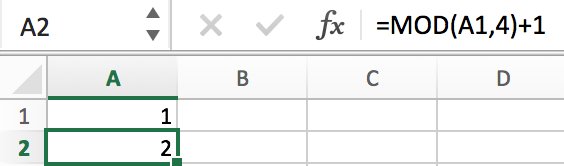Step 2: Drag A2 to A3. Here the formula is updated to ‘=MOD(A2,4)+1’, as A2=2 is still smaller than 4, so MOD(A2,4)=2, in A3 cell, we get 3.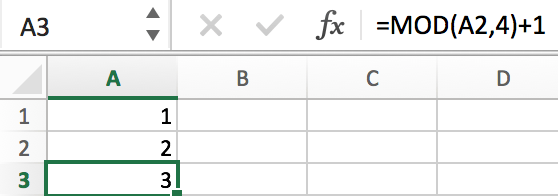Step 3: Execute the same way for A4. And when we drag A4 to A5, we can find that we get 1 again. That’s because use the function “=MOD(A4,4)” we can get 0 after calculation, so A5=MOD(A4,4)+1=1 again.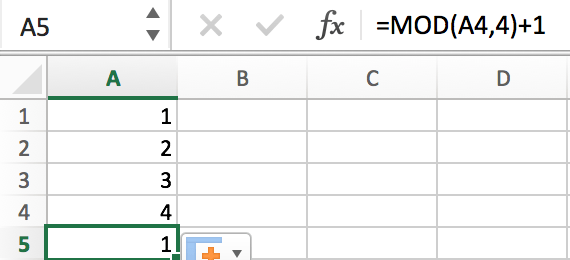Step 4: Fill A6-A12 for example. Then we get three groups of numbers 1,2,3,4.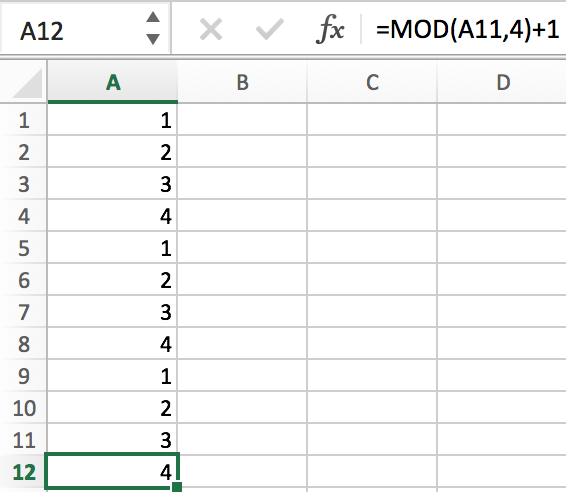Notes: this way is only valuable for simple number sequence. And the started number should be 1.

Sidebar